# Difference between revisions of "0708-1300/the unit sphere in a Hilbert space is contractible"

Let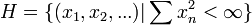$H=\{(x_1,x_2,...)| \sum x_n^2<\infty\}$ and define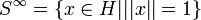$S^{\infty}=\{x\in H| ||x||=1\}$

Claim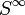$S^{\infty}$ is contractible

Proof

Suppose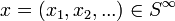$x=(x_1,x_2,...)\in S^{\infty}$ then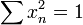$\sum x_n^2=1$

Define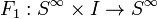$F_1:S^{\infty}\times I\rightarrow S^{\infty}$ by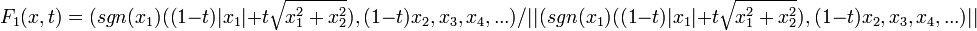$F_1(x,t)=(sgn(x_1)((1-t)|x_1|+t\sqrt{x_1^2+x_2^2}),(1-t)x_2,x_3,x_4,...)/||(sgn(x_1)((1-t)|x_1|+t\sqrt{x_1^2+x_2^2}),(1-t)x_2,x_3,x_4,...)||$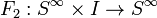$F_2:S^{\infty}\times I\rightarrow S^{\infty}$ by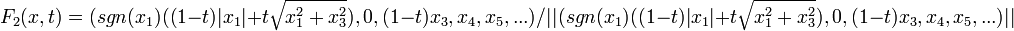$F_2(x,t)=(sgn(x_1)((1-t)|x_1|+t\sqrt{x_1^2+x_3^2}),0,(1-t)x_3,x_4,x_5,...)/||(sgn(x_1)((1-t)|x_1|+t\sqrt{x_1^2+x_3^2}),0,(1-t)x_3,x_4,x_5,...)||$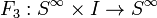$F_3:S^{\infty}\times I\rightarrow S^{\infty}$ by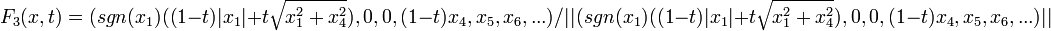$F_3(x,t)=(sgn(x_1)((1-t)|x_1|+t\sqrt{x_1^2+x_4^2}),0,0,(1-t)x_4,x_5,x_6,...)/||(sgn(x_1)((1-t)|x_1|+t\sqrt{x_1^2+x_4^2}),0,0,(1-t)x_4,x_5,x_6,...)||$

and so on ...

applying the homotopy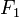$F_1$ in the time interval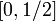$[0,1/2]$,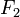$F_2$ in the interval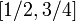$[1/2,3/4]$,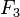$F_3$ in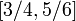$[3/4,5/6]$ etc...

we get the desired contraction to the point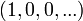$(1,0,0,...)$.

A different way to see this is via the cellular structure of$S^{\infty}$. If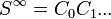$S^{\infty}=C_0 C_1 ...$ you can always contract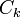$C_k$ along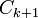$C_{k+1}$ like moving contracting the equator along the surface of the earth.